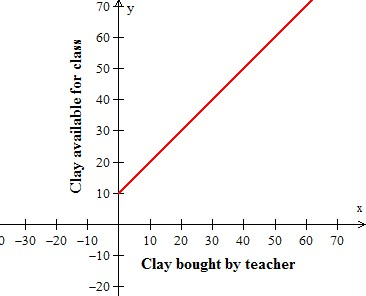# Identifying independent and dependent quantities from tables and graphs

#### Excel Pivot Tables and Dashboard. Simple & Quick tutorial!

Most Popular

7 Lectures 58 mins

#### Learn HTML Tables

15 Lectures 59 mins

#### Excel Analytics - Data Analysis with Pivot-Tables and Charts

46 Lectures 2.5 hours

Many real-world situations involve two variable quantities in which one quantity depends on the other. The quantity that depends on the other quantity is called the dependent variable, and the quantity it depends on is called the independent variable. The values of variables are used in tables and in plotting graphs.

In this lesson, we identify the dependent variable and the independent variable in a table or a graph.

Identify the dependent and independent variables in the following problem.### Solution

Independent variable is Clay bought by teacher; Dependent variable is Clay available for class

Identify the dependent and independent variables in the following problem.

Time in years versus the weight in pounds is shown in the table below.

 Time in years(x) Weighr in pounds(y) 0 1 2 3 0 60 120 180

### Solution

Independent variable is Time in years; Dependent variable is Weight in pounds.